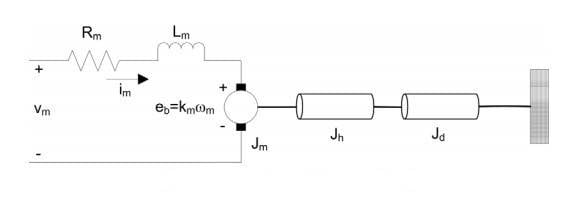# Control Transfer functions basics

• gl0ck

#### gl0ck

Hello,

I got the following diagram, shown below, and I have to derive its transfer function. I think I have a general misunderstanding about the transfer functions. What I think it is, is: output/input basically. As input is the whole block of things that affect the output.

This is the system that I have.## Homework Equations

After doing some thinking how to start this I came out with the following:
$$v_m ( t ) - R_m i_m ( t ) - L_m { \frac {\ di_m (t) } { dt}} -k_m ω _m ( t ) = 0$$
It was said that we could ignore the inductance as the motor resistance is much bigger so I came up with as solving for im(t)
$$i_m (t) = {\frac {v_m (t) - k_m ω_m (t) }{R_m}}$$
I am given also a expression of the motor shaft equation:
$$J_{eq} ω_m (t) = τ_m (t)$$ , given that the Jeq is the total moment inertia acting on the motor. Also the torque is given by $$τ_m = k_m i_m (t)$$

I also know that the Jeq = Jm + Jh + Jd
With Jm and Jh given in a separate table.
I've found Jd to be $$J_d = {\frac {1}{2}} m r^2$$

## The Attempt at a Solution

So far I've only seen transfer function of an electrical circuit like RC and RL circuits. As I am studying electrical engineering I am kind of confused how I am supposed to derive the transfer function with all this torque and angular velocity. I am asked to derive the transfer function of input voltage and the angular velocity.
I can see that I can substitute what I've found for im into the torque equation, but don't know how then I can turn that into the (s) domain.

Any guidance is much appreciated !
Thanks

Last edited:
I can see that I can substitute what I've found for I am into the torque equation, but don't know how then I can turn that into the (s) domain.

How do you go from the time domain to the s domain in a purely electrical problem??

also I might be wrong but it looks like there is an error with the equation you were given
wm is angular velocity.

Jeqωm(t)=τm(t) should be Jeqωm'(t)=τm(t) given that friction is 0.

also here is a handy guide that derives motor speed / armature voltage.
http://ctms.engin.umich.edu/CTMS/index.php?example=MotorSpeed&section=SystemModeling

note: you may be thinking of transfer functions in an odd way. Below is something to maybe help you understand?
I think I have a general misunderstanding about the transfer functions. What I think it is, is: output/input basically.

lets take a current to voltage circuit. your transfer function needs to have the units of voltage/current.

therefore...

lets look at an airplane control yoke.
you could say turning right on the yoke rolls the plane the the right, the higher the angle, the greater the roll.

so let's say our transfer function is P(Roll Rate)/angle

well yes, but there is more to it. you see moving the yoke might just turn a knob on a potentiometer.

so really we have

p=voltage/angle (the potentiometer transfer function) * P/voltage (the roll rate transfer function)

but no, because that has to go through some com protocol and acuator gain we call X

so

p = voltage/angle (potentiometer gain) * X/voltage (voltage to actuator gain) * P/X (roll rate gain)

we can continue this process for a while.

The essence of understanding transfer functions like this is to make sure the units work out. so if you want to get a transfer function of w/voltage (angluar velocity per volt), its really just a combination of different smaller transfer functions. break it down if you have to.

in the end, it is no different from a 5 stage voltage filter ( volt/volt * volt/volt * current/volt * volt/current *volt/volt)
at the end of the day, that is a volt/volt filter
understanding the units is the key to understanding controls!I don't know if you are familiar with Laplace?

You start from the left with:

1) Summation block: +Vm, -eb, output = Verr.

2) Find Im, multiplying Verr with 1/(Rm + sLm), output = Im

3) Find motor torque from Im

4) Find dω/dt from torque, inertia

5) Find ω by integration

6) Find eb and feed back to summation block 1)

Now use Mason's rule to calculate the transfer function of the system.

Thank you both for the answers. donpacino, I looked at the link it helped find the transfer function in my case. What I found more helpful actually was the comment below. I also found the book Modern Control Systems quite helpful understanding some basics.

This is a poorly presented problem. What transfer function are you looking for? And what is that thing on the right - just a frictionless bearing or?

I suspect you're looking for ωm/Vm. And the thing on the right IS just a frictionless bearing. If you can confirm we could proceed with help.